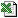Business Functions Library for Excel

Note: In order to use the spreadsheet examples, you need to download Business Functions
Depreciate (Time, Base, StartDep, LifeOrDecRate, DepType, InitialValue, [FinalValue], [DayCount], [Periods], [ProjMode])
Depreciate
Calculates depreciation for a time period.
Auto Array Function
###### Extra Features

DepType has to 0,1,2, or 3 to use one of the pre-defined depreciation types. However any other number is used as the factor for n-declining balance depreciation. See the example for more details

###### Discussion

There are two depreciation functions, DepreciatedValue and Depreciate, the first calculates the value of an asset at a specific point in time, and the second calculates the depreciation during a timeperiod. Depreciate works by simply determining the difference in the value of the asset between the beginning and end of the timeperiod. DepreciatedValue has a list of input parameters that entirely describe the assets life.

• The InitialDate is when the asset is purchased and comes into being in the accounts. It is not necessarily when the asset starts to depreciate.
• The InitialValue is the value at the initial date. The assets depreciated value stays at InitialValue until the asset"s value starts to depreciate.
• Depreciation starts on the date denoted by StartDep. This can, and often is, the same as InitialDate, meaning tha depreciation starts immediately. But with this function depreciateion does not have to start immediately.
• Once depreciation starts, the way that the value of the asset declines is determined by LifeOrDecRate and DepType . The LifeOrDecRate parameter works in one of two ways. If you specify an annual rate, as a fraction less than 1 (eg 0.25 for 25% per year), this is the rate of depreciation that is applied, whether its Straight-Line, Declining or DoubleDeclining. If you specify a number greater or equal to 1, then this is used as the lifetime over which the asset is depreciated. Note that the life commences at the start of depreciation, StartDep, not InitialDate. Therefore, LifeOrDecRate=0.25, and LifeOrDecRate=4 amount to the same thing for straight line depreciation. Note that for the Sum Of Years digits type depreciation the life must boild down to and integer number of years.
• DepType determines the TYPE Of depreciation applied between StartDep, when the asset starts to be depreciated, and the end of the assets life as defined by LifeOrDecRate. You can also optionally set a FinalValue, so that the asset value does not have to decline to zero, but perhaps to some other salvage value. The four types of depreciation currently supported and well-documeneted in the literature, they are STRAIGHT-LINE (the value of the asset declines linearly over time), DECLINING (the value declines exponentially, for example each year the asset declines by 25%, or is 75% of its previous years value), DOUBLE-DECLINING (discussed below), and SUM OF YEARS DIGITS.
• Double-declining depreciation is where the value declines according to a logarithmic relationship according to an input factor "f". The way you set factor "f", is to set DepType, as this factor. The function knows that any value other than zero, 1, or 3 for DepType MUST be the factor "f" for double-declining balance type depreciation. Once f is determined the RATE of decline is determined by using LifeOrDecRate so that the decline rate is equal to the factor divided by LifeOrDecRate (if LifeOrDecRate refers to the life in years of the asset). If LifeOrDecRate refers to a rate of decline, then the life of the asset is determined as factor "f" divided by this life in years. Once the double-declining rate is established, the value at any point in time is: exp(log(InitialValue)+log(1-rate)*t), where t is the number of years elapsed since depreciation began.
• To recap in overall terms, the depreciation functions model the asset, initially with a zero value, coming into being at time InitialDate. the value of the asset stays at InitialValue, whereupon it starts to depreciate according to DepType and LifeOrDecRate. Depreciation carries on until the assets value reaches zero or the optional FinalValue.
• Note that the asset will STAY at the the value of FinalValue indefinitely - it is the lowermost value this asset can ever have. If you have a non-zero FinalValue and wish to "cut off" the depreciated value, then the best thing to do is simply wrap your DepreciatedValue function with a Level function which will just return zero if outside its dates limits, for example: Level(TheDate,InitialDate,MyCutOffDate,DepreciatedValue(TheDate,...)).

###### ExampleDepreciate.xls
###### Templatesdepreciation.xlsdepreciation_schedule.xls